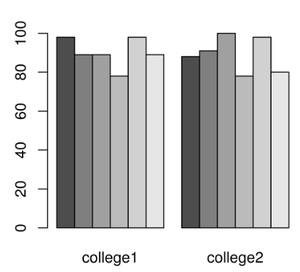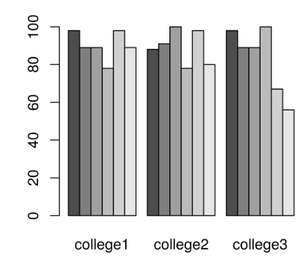GeeksforGeeks App
Open AppBrowser
Continue

# Create multiple barplots in R side by side

In R programming language, barplot is a graphical representation of linear data which is one-dimensional. Bar plot is used for statistical analysis for easy understanding of the data. It represents the given data in the form of bars. It can give bar graphs along x-axis and y-axis. Where x-axis represents the name of the data and y-axis represents the bar graph values from 0 to n range.

In this article, we are going to create multiple bar plots side by side in R Programming. If we want to create multiple bar plots side by side then we have to specify the parameter in the above syntax i.e. beside=T. It will specify True. This will place the second, third, ., so on to the next of the bar plots

Syntax:

barplot(data,beside=T)

Where, beside is to place bar plots side by side

Hence, to draw multiple barplots side by side the data for each barplot is initialized and combined using cbind(). Then it is actually plotted using barplot() with beside set to TRUE(T).

Example 1:

## R

 `# college1 vector``college1=``c``(98,89,89.0,78,98,89)`` ` `# college2 vector``college2=``c``(88,91,100,78,98,80)`` ` `# combine two vectors using cbind ``# function``college_data=``cbind``(college1,college2)`` ` `# pass this college_data to the ``# barplot``barplot``(college_data,beside=T)`

Output:Example 2:

## R

 `# college1 vector``college1=``c``(98,89,89.0,78,98,89)`` ` `# college2 vector``college2=``c``(88,91,100,78,98,80)`` ` `# college3 vector``college3=``c``(98,89,89.0,100,67,56)`` ` ` ` `# combine three college vectors ``# using cbind function``college_data=``cbind``(college1,college2,college3)`` ` `# pass this college_data to the barplot``barplot``(college_data,beside=T)`

Output:My Personal Notes arrow_drop_up